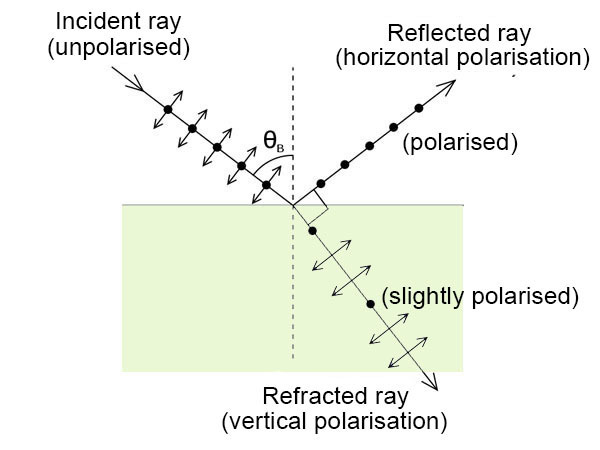# Brewster's Angle Calculator

This is an online calculator that calculates Brewster's Angle. Just enter the Refractive Index of the Incident Medium and Refractive Index of the Refracted Medium to get the corresponding Brewster's Angle value.

### Result

• Brewster

Brewster’s angle (or polarization angle) is the angle of incidence at which an unpolarized light that contains both horizontal and vertical polarization separates into a vertically and a horizontally polarized wave. When a ray of light is incident on a surface of two different refractive indices, the ray is partially reflected and partially transmitted.

When the incident angle is equal to Brewster’s angle, the wave which is transmitted through the other medium contains only the vertical polarization and the reflected light coming out of the surface contains only the horizontal polarization, i.e. we obtain a plane polarized light.

It is given by,Here n1 is the refractive index of the incident medium and n2 is the refractive index of the refractive medium.Natural light contains both horizontal and vertical polarizations. Polarization is nothing but the direction of the electric field present in the electromagnetic wave or the light. In an unpolarized light, the electric field lines are randomly oriented and do not have a specific direction of oscillation.

When incident at the surface of medias with different refractive indices at an incident angle equal to the brewster’s angle, the reflected light is observed to be plane polarized. This means that the electric field vibrations in the reflected light are only in a single direction (usually horizontal).

When a ray of light is incident on a surface, the ray is partially reflected and partially transmitted. When we increase the incident angle, the reflected and transmitted angle also changes. At a point where the reflected ray and the transmitted ray are perpendicular to each other, it is found that the reflected ray is completely plane polarized. This angle of incidence at which the reflected and the transmitted rays are at right angles to each other so that the reflected ray contains only a single plane of polarization is known as the brewster’s angle.

Applying Snell’s law to the interface of the two media, we get,Here θi and θt are the incident and the transmitted angles respectively.

At brewster’s angle, the reflected ray and the transmitter ray are at right angles, i.e; θ+ θt = 90˚, where θt is the transmitted angle.

We can rewrite this as, θt = 90˚ - θ

We know from the law of reflection, θi = θr. So we get, θt = 90˚ - θi

So at brewster’s angle, (1) can be rewritten as,